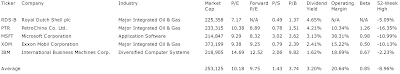## Pages

### 5 Mega Caps And Their Dividends

Dividends From Mega Capitalized Stocks by Dividend Yield - Stock, Capital, Investment. Here is a current sheet of stocks with a market capitalization of more than USD 200 billion (mega caps) and a positive dividend yield. 5 stocks and one non-dividend paying stock (Apple – AAPL) fulfilled these criteria of which 2 yielding above 4 percent. Exxon Mobil (XOM) is unbeaten the number one. The average market capitalization amounts to USD 253 billion.

Here are the 3 top dividend stocks by dividend yield:

Royal Dutch Shell (NYSE:RDS.A) has a market capitalization of \$219.21 billion. The company employs 97,000 people, generates revenues of \$368,056.00 million and has a net income of \$20,474.00 million. The firm’s earnings before interest, taxes, depreciation and amortization (EBITDA) amounts to \$42,320.00 million. Because of these figures, the EBITDA margin is 11.50 percent (operating margin 8.75 percent and the net profit margin finally 5.56 percent).

The total debt representing 13.74 percent of the company’s assets and the total debt in relation to the equity amounts to 29.95 percent. Due to the financial situation, the return on equity amounts to 14.15 percent. Finally, earnings per share amounts to \$10.07 of which \$3.36 were paid in form of dividends to shareholders last fiscal.

Here are the price ratios of the company: The P/E ratio is 6.90, Price/Sales 0.62 and Price/Book ratio 1.47. Dividend Yield: 4.76 percent. The beta ratio is 1.03.Long-Term Stock Chart Of Royal Dutch Shell plc ... (Click to enlarge)

PetroChina (NYSE:PTR) has a market capitalization of \$233.32 billion. The company employs 552,698 people, generates revenues of \$230,643.30 million and has a net income of \$23,733.32 million. The firm’s earnings before interest, taxes, depreciation and amortization (EBITDA) amounts to \$48,189.37 million. Because of these figures, the EBITDA margin is 20.89 percent (operating margin 12.81 percent and the net profit margin finally 10.29 percent).

The total debt representing 14.10 percent of the company’s assets and the total debt in relation to the equity amounts to 24.88 percent. Due to the financial situation, the return on equity amounts to 15.68 percent. Finally, earnings per share amounts to \$12.27 of which \$5.42 were paid in form of dividends to shareholders last fiscal.

Here are the price ratios of the company: The P/E ratio is 10.39, Price/Sales 1.25 and Price/Book ratio 1.66. Dividend Yield: 4.00 percent. The beta ratio is 1.26.Long-Term Stock Chart Of PetroChina Co. Ltd. (ADR) (Click to enlarge)

Microsoft Corporation (NASDAQ:MSFT) has a market capitalization of \$214.85 billion. The company employs 90,000 people, generates revenues of \$69,943.00 million and has a net income of \$23,150.00 million. The firm’s earnings before interest, taxes, depreciation and amortization (EBITDA) amounts to \$29,927.00 million. Because of these figures, the EBITDA margin is 42.79 percent (operating margin 38.83 percent and the net profit margin finally 33.10 percent).

The total debt representing 10.97 percent of the company’s assets and the total debt in relation to the equity amounts to 20.88 percent. Due to the financial situation, the return on equity amounts to 44.84 percent. Finally, earnings per share amounts to \$2.75 of which \$0.64 were paid in form of dividends to shareholders last fiscal.

Here are the price ratios of the company: The P/E ratio is 9.27, Price/Sales 3.22 and Price/Book ratio 3.93. Dividend Yield: 2.99 percent. The beta ratio is 0.99.Long-Term Stock Chart Of Microsoft Corporation (Click to enlarge)

Here is the full table with some fundamentals (TTM):5 Megacaps And Their Dividends (Click to enlarge)

Take a closer look at the full table of mega caps. The average price to earnings ratio (P/E ratio) amounts to 10.2 while the forward price to earnings ratio is 9.8. The dividend yield has a value of 3.2 percent. Price to book ratio is 3.7 and price to sales ratio 1.4. The operating margin amounts to 20.6 percent.

Related stock ticker symbols:
RDS-B, PTR, MSFT, XOM, IBM

Selected Articles: